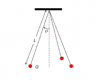# Three hanging balls of uniformly distributed charge

• derravaragh

## Homework Statement

Three identical small balls of mass m with uniformly distributed charge Q each hang from a string of length L. The strings are all tethered at the opposite end at the same point.
(a) Find the general equation for the angle θ of each string from vertical.

## The Attempt at a Solution

I started off by drawing a free body diagram, obtaining a Tension in both the x and y directions, a downward force (m*g) and a horizontal force away in the x-direction (the force due to the other charges). I then collected the Forces for the x and y directions:
Fy = Tcos(θ) - mg = 0
Fx = Tsin(θ) - Q2/(3*L2*sin2(θ))

Using Fy I solve for T to be mg/cos(θ) and plug it in for T in Fx. However, a problem arises when I try to solve for θ, I end up with:

tan(θ)sin2(θ) = Q2/(3L2mg)

I do not know how to solve this for θ, and when re-evaluating my work, I feel my value for the distance between balls in the force due to a charge is incorrect. The balls make a triangle, and with the invisible line down the center, you can divide that triangle into three even 30-30-120 triangles. I determined the distance between one ball and the center to be Lsin(θ), and using the Law of Sines, determined r (the distance between two balls) to be:

r = √(3)*L*sin(θ)

If this is correct, I'm not sure where I went wrong. Any hints would be appreciated. Thanks.

#### Attachments

•problem 3.png
1.6 KB · Views: 391

## Homework Statement

Three identical small balls of mass m with uniformly distributed charge Q each hang from a string of length L. The strings are all tethered at the opposite end at the same point.
(a) Find the general equation for the angle θ of each string from vertical.

## The Attempt at a Solution

I started off by drawing a free body diagram, obtaining a Tension in both the x and y directions, a downward force (m*g) and a horizontal force away in the x-direction (the force due to the other charges). I then collected the Forces for the x and y directions:
Fy = Tcos(θ) - mg = 0
Fx = Tsin(θ) - Q2/(3*L2*sin2(θ))

Using Fy I solve for T to be mg/cos(θ) and plug it in for T in Fx. However, a problem arises when I try to solve for θ, I end up with:

tan(θ)sin2(θ) = Q2/(3L2mg)

I do not know how to solve this for θ, and when re-evaluating my work, I feel my value for the distance between balls in the force due to a charge is incorrect. The balls make a triangle, and with the invisible line down the center, you can divide that triangle into three even 30-30-120 triangles. I determined the distance between one ball and the center to be Lsin(θ), and using the Law of Sines, determined r (the distance between two balls) to be:

r = √(3)*L*sin(θ)

If this is correct, I'm not sure where I went wrong. Any hints would be appreciated. Thanks.

## The Attempt at a Solution

Your equations for r is correct. The force on a charge is the vector sum of the Coulomb forces from the other two charges. Take that into account. And do not forget the factor k from the Coulomb force!

At the end, you get a third -order equation for tanθ, replacing sin2θ by tan2θ/(1+tan2θ)

ehild